# Survival regression¶

Often we have additional data aside from the duration that we want to use. The technique is called survival regression – the name implies we regress covariates (e.g., age, country, etc.) against another variable – in this case durations. Similar to the logic in the first part of this tutorial, we cannot use traditional methods like linear regression because of censoring.

There are a few popular models in survival regression: Cox’s model, accelerated failure models, and Aalen’s additive model. All models attempt to represent the hazard rate $$h(t | x)$$ as a function of $$t$$ and some covariates $$x$$. We explore these models next.

## The dataset for regression¶

The dataset required for survival regression must be in the format of a Pandas DataFrame. Each row of the DataFrame represents an observation. There should be a column denoting the durations of the observations. There may (or may not) be a column denoting the event status of each observation (1 if event occurred, 0 if censored). There are also the additional covariates you wish to regress against. Optionally, there could be columns in the DataFrame that are used for stratification, weights, and clusters which will be discussed later in this tutorial.

An example dataset we will use is the Rossi recidivism dataset, available in lifelines as load_rossi().

from lifelines.datasets import load_rossi

"""
week  arrest  fin  age  race  wexp  mar  paro  prio
0      20       1    0   27     1     0    0     1     3
1      17       1    0   18     1     0    0     1     8
2      25       1    0   19     0     1    0     1    13
3      52       0    1   23     1     1    1     1     1
"""


The DataFrame rossi contains 432 observations. The week column is the duration, the arrest column denotes if the event (a re-arrest) occurred, and the other columns represent variables we wish to regress against.

## Cox’s proportional hazard model¶

The idea behind Cox’s proportional hazard model is that the log-hazard of an individual is a linear function of their covariates and a population-level baseline hazard that changes over time. Mathematically:

$\underbrace{h(t | x)}_{\text{hazard}} = \overbrace{b_0(t)}^{\text{baseline hazard}} \underbrace{\exp \overbrace{\left(\sum_{i=1}^n b_i (x_i - \overline{x_i})\right)}^{\text{log-partial hazard}}}_ {\text{partial hazard}}$

Note a few behaviors about this model: the only time component is in the baseline hazard, $$b_0(t)$$. In the above equation, the partial hazard is a time-invariant scalar factor that only increases or decreases the baseline hazard. Thus changes in covariates will only inflate or deflate the baseline hazard.

Note

In other regression models, a column of 1s might be added that represents that intercept or baseline. This is not necessary in the Cox model. In fact, there is no intercept in the Cox model - the baseline hazard represents this. lifelines will throw warnings and may experience convergence errors if a column of 1s is present in your dataset or formula.

### Fitting the regression¶

The implementation of the Cox model in lifelines is under CoxPHFitter. We fit the model to the dataset using fit(). It has a print_summary() function that prints a tabular view of coefficients and related stats.

from lifelines import CoxPHFitter

cph = CoxPHFitter()
cph.fit(rossi, duration_col='week', event_col='arrest')

cph.print_summary()  # access the individual results using cph.summary

"""
<lifelines.CoxPHFitter: fitted with 432 total observations, 318 right-censored observations>
duration col = 'week'
event col = 'arrest'
number of observations = 432
number of events observed = 114
partial log-likelihood = -658.75
time fit was run = 2019-10-05 14:24:44 UTC

---
coef  exp(coef)   se(coef)   coef lower 95%   coef upper 95%  exp(coef) lower 95%  exp(coef) upper 95%
fin   -0.38       0.68       0.19            -0.75            -0.00                 0.47                 1.00
age   -0.06       0.94       0.02            -0.10            -0.01                 0.90                 0.99
race   0.31       1.37       0.31            -0.29             0.92                 0.75                 2.50
wexp  -0.15       0.86       0.21            -0.57             0.27                 0.57                 1.30
mar   -0.43       0.65       0.38            -1.18             0.31                 0.31                 1.37
paro  -0.08       0.92       0.20            -0.47             0.30                 0.63                 1.35
prio   0.09       1.10       0.03             0.04             0.15                 1.04                 1.16

z      p   -log2(p)
fin  -1.98   0.05       4.40
age  -2.61   0.01       6.79
race  1.02   0.31       1.70
wexp -0.71   0.48       1.06
mar  -1.14   0.26       1.97
paro -0.43   0.66       0.59
prio  3.19 <0.005       9.48
---
Concordance = 0.64
Partial AIC = 1331.50
log-likelihood ratio test = 33.27 on 7 df
-log2(p) of ll-ratio test = 15.37
"""


New in v0.25.0, We can also use ✨formulas✨ to handle the right-hand-side of the linear model. For example:

cph.fit(rossi, duration_col='week', event_col='arrest', formula="fin + wexp + age * prio")


is analgous to the linear model with interaction term:

$\beta_1\text{fin} + \beta_2\text{wexp} + \beta_3 \text{age} + \beta_4 \text{prio} + \beta_5 \text{age} \cdot \text{prio}$
cph.fit(rossi, duration_col='week', event_col='arrest', formula="fin + wexp + age * prio")
cph.print_summary()

"""
<lifelines.CoxPHFitter: fitted with 432 total observations, 318 right-censored observations>
duration col = 'week'
event col = 'arrest'
baseline estimation = breslow
number of observations = 432
number of events observed = 114
partial log-likelihood = -659.39
time fit was run = 2020-07-13 19:30:33 UTC

---
coef  exp(coef)   se(coef)   coef lower 95%   coef upper 95%  exp(coef) lower 95%  exp(coef) upper 95%
covariate
fin        -0.33       0.72       0.19            -0.70             0.04                 0.49                 1.05
wexp       -0.24       0.79       0.21            -0.65             0.17                 0.52                 1.19
age        -0.03       0.97       0.03            -0.09             0.03                 0.92                 1.03
prio        0.31       1.36       0.17            -0.03             0.64                 0.97                 1.90
age:prio   -0.01       0.99       0.01            -0.02             0.01                 0.98                 1.01

z    p   -log2(p)
covariate
fin       -1.73 0.08       3.57
wexp      -1.14 0.26       1.97
age       -0.93 0.35       1.51
prio       1.80 0.07       3.80
age:prio  -1.28 0.20       2.32
---
Concordance = 0.64
Partial AIC = 1328.77
log-likelihood ratio test = 31.99 on 5 df
-log2(p) of ll-ratio test = 17.35
"""


Formulas can be used to create interactions, encode categorical variables, create basis splines, and so on. The formulas used are (almost) the same as what’s available in R and statsmodels.

### Interpretation¶

To access the coefficients and the baseline hazard directly, you can use params_ and baseline_hazard_ respectively. Taking a look at these coefficients for a moment, prio (the number of prior arrests) has a coefficient of about 0.09. Thus, a one unit increase in prio means the the baseline hazard will increase by a factor of $$\exp{(0.09)} = 1.10$$ - about a 10% increase. Recall, in the Cox proportional hazard model, a higher hazard means more at risk of the event occurring. The value $$\exp{(0.09)}$$ is called the hazard ratio, a name that will be clear with another example.

Consider the coefficient of mar (whether the subject is married or not). The values in the column are binary: 0 or 1, representing either unmarried or married. The value of the coefficient associated with mar, $$\exp{(-.43)}$$, is the value of ratio of hazards associated with being married, that is:

$\exp(-0.43) = \frac{\text{hazard of married subjects at time t}}{\text{hazard of unmarried subjects at time t}}$

Note that left-hand side is a constant (specifically, it’s independent of time, $$t$$), but the right-hand side has two factors that may vary with time. The proportional hazard assumption is that relationship is true. That is, hazards can change over time, but their ratio between levels remains a constant. Later we will deal with checking this assumption. However, in reality, it’s very common for the hazard ratio to change over the study duration. The hazard ratio then has the interpretation of some sort of weighted average of period-specific hazard ratios. As a result, the hazard ratio may critically depend on the duration of the follow-up.

### Convergence¶

Fitting the Cox model to the data involves using iterative methods. lifelines takes extra effort to help with convergence, so please be attentive to any warnings that appear. Fixing any warnings will generally help convergence and decrease the number of iterative steps required. If you wish to see more information during fitting, there is a show_progress parameter in fit() function. For further help, see Problems with convergence in the Cox proportional hazard model.

After fitting, the value of the maximum log-likelihood this available using log_likelihood_. The variance matrix of the coefficients is available under variance_matrix_.

### Goodness of fit¶

After fitting, you may want to know how “good” of a fit your model was to the data. A few methods the author has found useful is to

### Prediction¶

After fitting, you can use use the suite of prediction methods: predict_partial_hazard(), predict_survival_function(), and others. See also the section on Predicting censored subjects below

X = rossi

cph.predict_survival_function(X)
cph.predict_median(X)
cph.predict_partial_hazard(X)
...


### Penalties and sparse regression¶

It’s possible to add a penalizer term to the Cox regression as well. One can use these to i) stabilize the coefficients, ii) shrink the estimates to 0, iii) encourages a Bayesian viewpoint, and iv) create sparse coefficients. All regression models, including the Cox model, include both an L1 and L2 penalty:

$\frac{1}{2} \text{penalizer} \left((1-\text{l1-ratio}) \cdot ||\beta||_2^2 + \text{l1-ratio} \cdot ||\beta||_1\right)$

Note

It’s not clear from the above, but intercept (when applicable) are not penalized.

To use this in lifelines, both the penalizer and l1_ratio can be specified in the class creation:

from lifelines import CoxPHFitter

cph = CoxPHFitter(penalizer=0.1, l1_ratio=1.0) # sparse solutions,
cph.fit(rossi, 'week', 'arrest')
cph.print_summary()


Instead of a float, an array can be provided that is the same size as the number of penalized parameters. The values in the array are specific penalty coefficients for each covariate. This is useful for more complicated covariate structure. Some examples:

1. you have lots of confounders you wish to penalizer, but not the main treatment(s).
from lifelines import CoxPHFitter

# variable fin is the treatment of interest so don't penalize it at all
penalty = np.array([0, 0.5, 0.5, 0.5, 0.5, 0.5, 0.5])

cph = CoxPHFitter(penalizer=penalty)
cph.fit(rossi, 'week', 'arrest')
cph.print_summary()

1. you have to fuse categories together.
2. you want to implement a very sparse solution.

See more about penalties and their implementation on our development blog.

### Plotting the coefficients¶

With a fitted model, an alternative way to view the coefficients and their ranges is to use the plot method.

from lifelines.datasets import load_rossi
from lifelines import CoxPHFitter

cph = CoxPHFitter()
cph.fit(rossi, duration_col='week', event_col='arrest')

cph.plot()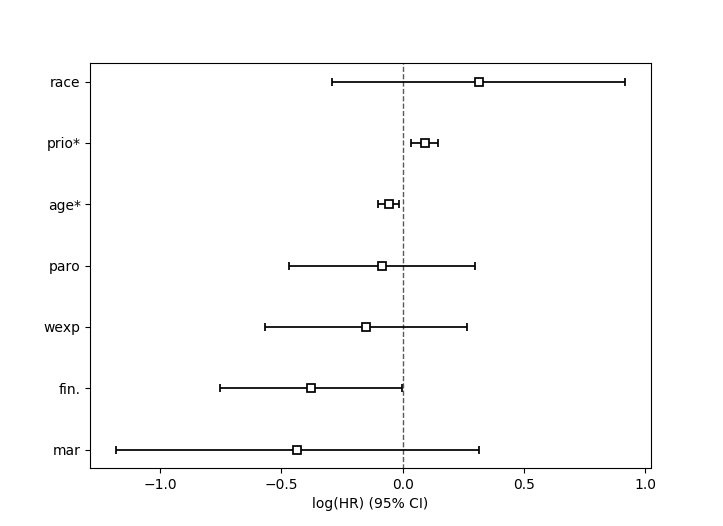### Plotting the effect of varying a covariate¶

After fitting, we can plot what the survival curves look like as we vary a single covariate while holding everything else equal. This is useful to understand the impact of a covariate, given the model. To do this, we use the plot_partial_effects_on_outcome() method and give it the covariate of interest, and the values to display.

Note

Prior to lifelines v0.25.0, this method used to be called plot_covariate_groups. It’s been renamed to plot_partial_effects_on_outcome (a much clearer name, I hope).

from lifelines.datasets import load_rossi
from lifelines import CoxPHFitter

cph = CoxPHFitter()
cph.fit(rossi, duration_col='week', event_col='arrest')

cph.plot_partial_effects_on_outcome(covariates='prio', values=[0, 2, 4, 6, 8, 10], cmap='coolwarm')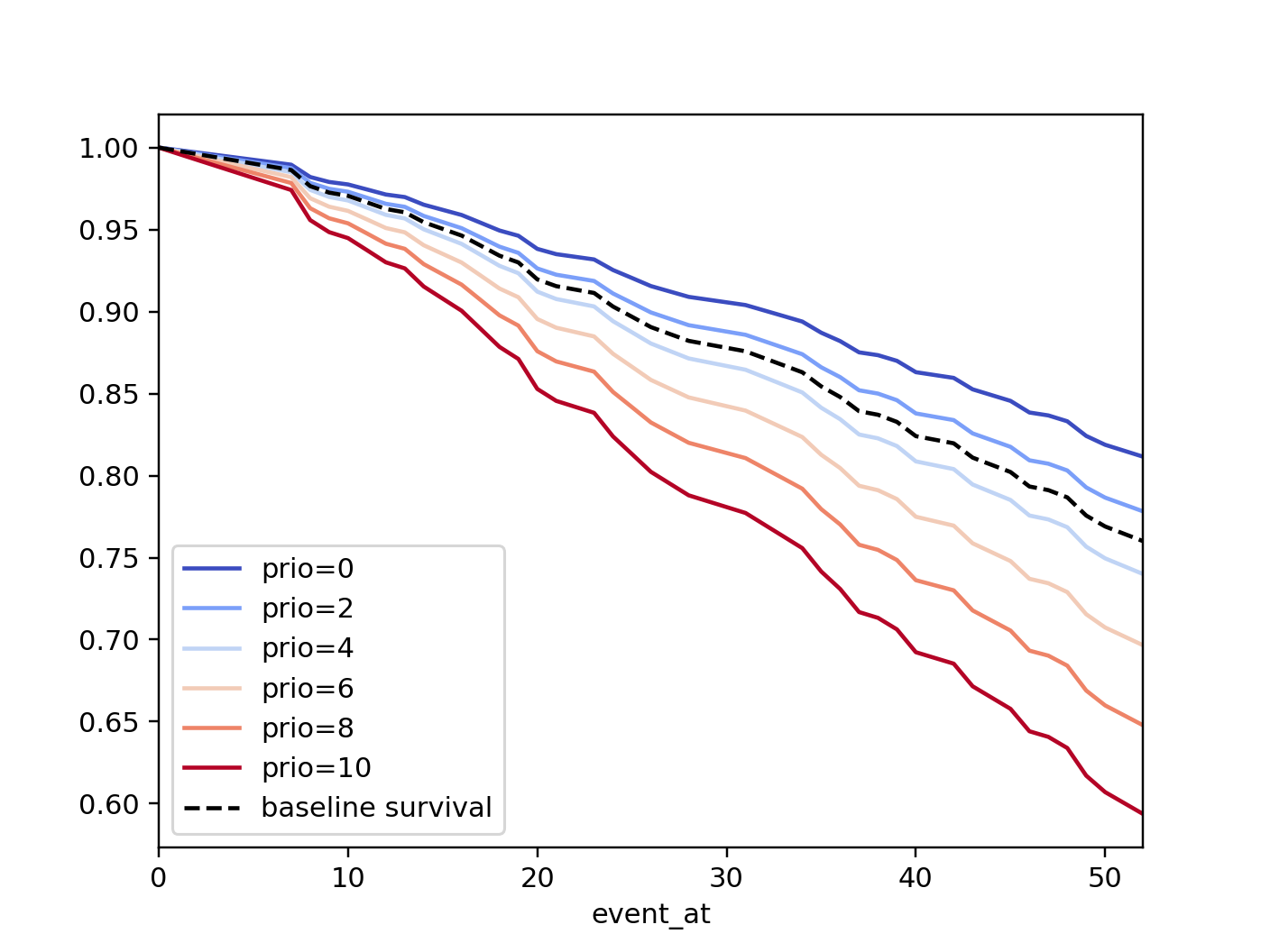If there are derivative features in your dataset, for example, suppose you have included prio and prio**2 in your dataset. It doesn’t make sense to just vary year and leave year**2 fixed. You’ll need to specify manually the values the covariates take on in a N-d array or list (where N is the number of covariates being varied.)

rossi['prio**2'] = rossi['prio'] ** 2

cph.fit(rossi, 'week', 'arrest')

cph.plot_partial_effects_on_outcome(
covariates=['prio', 'prio**2'],
values=[
[0, 0],
[1, 1],
[2, 4],
[3, 9],
[8, 64],
],
cmap='coolwarm')


However, if you used the formula kwarg in fit, all the necessary transformations will be made internally for you.

cph.fit(rossi, 'week', 'arrest', formula="prio + I(prio**2)")

cph.plot_partial_effects_on_outcome(
covariates=['prio'],
values=[0, 1, 2, 3, 8],
cmap='coolwarm')


This feature is also useful for analyzing categorical variables:

cph.plot_partial_effects_on_outcome(
covariates=["a_categorical_variable"]
values=["A", "B", ...],
plot_baseline=False)


### Checking the proportional hazards assumption¶

To make proper inferences, we should ask if our Cox model is appropriate for our dataset. Recall from above that when using the Cox model, we are implicitly applying the proportional hazard assumption. We should ask, does our dataset obey this assumption?

CoxPHFitter has a check_assumptions() method that will output violations of the proportional hazard assumption. For a tutorial on how to fix violations, see Testing the Proportional Hazard Assumptions. Suggestions are to look for ways to stratify a column (see docs below), or use a time varying model.

Note

Checking assumptions like this is only necessary if your goal is inference or correlation. That is, you wish to understand the influence of a covariate on the survival duration & outcome. If your goal is prediction, checking model assumptions is less important since your goal is to maximize an accuracy metric, and not learn about how the model is making that prediction.

### Stratification¶

Sometimes one or more covariates may not obey the proportional hazard assumption. In this case, we can allow the covariate(s) to still be including in the model without estimating its effect. This is called stratification. At a high level, think of it as splitting the dataset into m smaller datasets, partitioned by the unique values of the stratifying covariate(s). Each dataset has its own baseline hazard (the non-parametric part of the model), but they all share the regression parameters (the parametric part of the model). Since covariates are the same within each dataset, there is no regression parameter for the covariates stratified on, hence they will not show up in the output. However there will be m baseline hazards under baseline_cumulative_hazard_.

To specify variables to be used in stratification, we define them in the call to fit():

from lifelines.datasets import load_rossi
from lifelines import CoxPHFitter

cph = CoxPHFitter()
cph.fit(rossi, 'week', event_col='arrest', strata=['wexp'])
cph.print_summary()

"""
<lifelines.CoxPHFitter: fitted with 432 total observations, 318 right-censored observations>
duration col = 'week'
event col = 'arrest'
strata = ['wexp']
baseline estimation = breslow
number of observations = 432
number of events observed = 114
partial log-likelihood = -580.89
time fit was run = 2020-08-09 21:25:37 UTC

---
coef  exp(coef)   se(coef)   coef lower 95%   coef upper 95%  exp(coef) lower 95%  exp(coef) upper 95%
covariate
fin        -0.38       0.68       0.19            -0.76            -0.01                 0.47                 0.99
age        -0.06       0.94       0.02            -0.10            -0.01                 0.90                 0.99
race        0.31       1.36       0.31            -0.30             0.91                 0.74                 2.49
mar        -0.45       0.64       0.38            -1.20             0.29                 0.30                 1.34
paro       -0.08       0.92       0.20            -0.47             0.30                 0.63                 1.35
prio        0.09       1.09       0.03             0.03             0.15                 1.04                 1.16
z      p   -log2(p)
covariate
fin       -1.99   0.05       4.42
age       -2.64   0.01       6.91
race       1.00   0.32       1.65
mar       -1.19   0.23       2.09
paro      -0.42   0.67       0.57
prio       3.16 <0.005       9.33
---
Concordance = 0.61
Partial AIC = 1173.77
log-likelihood ratio test = 23.77 on 6 df
-log2(p) of ll-ratio test = 10.77

"""

cph.baseline_survival_.shape
# (49, 2)
cph.baseline_cumulative_hazard_.plot(drawstyle="steps")


### Weights & robust errors¶

Observations can come with weights, as well. These weights may be integer values representing some commonly occurring observation, or they may be float values representing some sampling weights (ex: inverse probability weights). In the fit() method, an kwarg is present for specifying which column in the DataFrame should be used as weights, ex: CoxPHFitter(df, 'T', 'E', weights_col='weights').

When using sampling weights, it’s correct to also change the standard error calculations. That is done by turning on the robust flag in fit(). Internally, CoxPHFitter will use the sandwich estimator to compute the errors.

import pandas as pd
from lifelines import CoxPHFitter

df = pd.DataFrame({
'T': [5, 3, 9, 8, 7, 4, 4, 3, 2, 5, 6, 7],
'E': [1, 1, 1, 1, 1, 1, 0, 0, 1, 1, 1, 0],
'weights': [1.1, 0.5, 2.0, 1.6, 1.2, 4.3, 1.4, 4.5, 3.0, 3.2, 0.4, 6.2],
'month': [10, 3, 9, 8, 7, 4, 4, 3, 2, 5, 6, 7],
'age': [4, 3, 9, 8, 7, 4, 4, 3, 2, 5, 6, 7],
})

cph = CoxPHFitter()
cph.fit(df, 'T', 'E', weights_col='weights', robust=True)
cph.print_summary()


See more examples in Adding weights to observations in a Cox model.

### Clusters & correlations¶

Another property your dataset may have is groups of related subjects. This could be caused by:

• a single individual having multiple occurrences, and hence showing up in the dataset more than once.
• subjects that share some common property, like members of the same family or being matched on propensity scores.

We call these grouped subjects “clusters”, and assume they are designated by some column in the DataFrame (example below). When using cluster, the point estimates of the model don’t change, but the standard errors will increase. An intuitive argument for this is that 100 observations on 100 individuals provide more information than 100 observations on 10 individuals (or clusters).

from lifelines import CoxPHFitter

df = pd.DataFrame({
'T': [5, 3, 9, 8, 7, 4, 4, 3, 2, 5, 6, 7],
'E': [1, 1, 1, 1, 1, 1, 0, 0, 1, 1, 1, 0],
'month': [10, 3, 9, 8, 7, 4, 4, 3, 2, 5, 6, 7],
'age': [4, 3, 9, 8, 7, 4, 4, 3, 2, 5, 6, 7],
'id': [1, 1, 1, 1, 2, 3, 3, 4, 4, 5, 6, 7]
})

cph = CoxPHFitter()
cph.fit(df, 'T', 'E', cluster_col='id')
cph.print_summary()


For more examples, see Correlations between subjects in a Cox model.

### Residuals¶

After fitting a Cox model, we can look back and compute important model residuals. These residuals can tell us about non-linearities not captured, violations of proportional hazards, and help us answer other useful modeling questions. See Assessing Cox model fit using residuals.

### Modeling baseline hazard and survival with parametric models¶

Normally, the Cox model is semi-parametric, which means that its baseline hazard, $$h_0(t)$$, has no parametric form. This is the default for lifelines. However, it is sometimes valuable to produce a parametric baseline instead. A parametric baseline makes survival predictions more efficient, allows for better understanding of baseline behaviour, and allows interpolation/extrapolation.

In lifelines, there is an option to fit to a parametric baseline with 1) cubic splines, or 2) piecewise constant hazards. Cubic splines are highly flexible and can capture the underlying data almost as well as non-parametric methods, and with much more efficiency.

from lifelines.datasets import load_rossi
from lifelines import CoxPHFitter

cph_spline = CoxPHFitter(baseline_estimation_method="spline", n_baseline_knots=5)
cph_spline.fit(rossi, 'week', event_col='arrest')


To access the baseline hazard and baseline survival, one can use baseline_hazard_ and baseline_survival_ respectively. One nice thing about parametric models is we can interpolate baseline survival / hazards too, see baseline_hazard_at_times() and baseline_survival_at_times().

Below we compare the non-parametric and the fully parametric baseline survivals:

cph_semi = CoxPHFitter().fit(rossi, 'week', event_col='arrest')
cph_piecewise = CoxPHFitter(baseline_estimation_method="piecewise", breakpoints=[20, 35]).fit(rossi, 'week', event_col='arrest')

bch_key = "baseline cumulative hazard"

ax = cph_spline.baseline_cumulative_hazard_[bch_key].plot(label="spline")
cph_semi.baseline_cumulative_hazard_[bch_key].plot(ax=ax, drawstyle="steps-post", label="semi")
cph_piecewise.baseline_cumulative_hazard_[bch_key].plot(ax=ax, label="peicewise[20,35]")
plt.legend()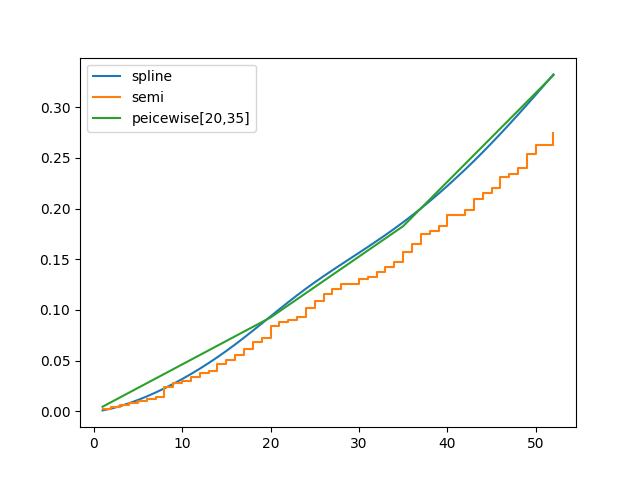Modeling the baseline survival with splines vs non-parametric.

lifelines’ spline Cox model can also use almost all the non-parametric options, including: strata, penalizer, timeline, formula, etc.

## Parametric survival models¶

We ended the previous section discussing a fully-parametric Cox model, but there are many many more parametric models to consider. Below we go over these, starting with the most common: AFT models.

### Accelerated failure time models¶

Suppose we have two populations, A and B, with different survival functions, $$S_A(t)$$ and $$S_B(t)$$, and they are related by some accelerated failure rate, $$\lambda$$:

$S_A(t) = S_B\left(\frac{t}{\lambda}\right)$

This can be interpreted as slowing down or speeding up moving along the survival function. A classic example of this is that dogs age at 7 times the rate of humans, i.e. $$\lambda = \frac{1}{7}$$. This model has some other nice properties: the average survival time of population B is $${\lambda}$$ times the average survival time of population A. Likewise with the median survival time.

More generally, we can model the $$\lambda$$ as a function of covariates available, that is:

$\begin{split}S_A(t) = S_B\left(\frac{t}{\lambda(x)}\right)\\ \lambda(x) = \exp\left(b_0 + \sum_{i=1}^n b_i x_i \right)\end{split}$

This model can accelerate or decelerate failure times depending on subjects’ covariates. Another nice feature of this is the ease of interpretation of the coefficients: a unit increase in $$x_i$$ means the average/median survival time changes by a factor of $$\exp(b_i)$$.

Note

An important note on interpretation: Suppose $$b_i$$ was positive, then the factor $$\exp(b_i)$$ is greater than 1, which will decelerate the event time since we divide time by the factor ⇿ increase mean/median survival. Hence, it will be a protective effect. Likewise, a negative $$b_i$$ will hasten the event time ⇿ reduce the mean/median survival time. This interpretation is opposite of how the sign influences event times in the Cox model! This is standard survival analysis convention.

Next, we pick a parametric form for the survival function, $$S(t)$$. The most common is the Weibull form. So if we assume the relationship above and a Weibull form, our hazard function is quite easy to write down:

$H(t; x) = \left( \frac{t}{\lambda(x)} \right)^\rho$

We call these accelerated failure time models, shortened often to just AFT models. Using lifelines, we can fit this model (and the unknown $$\rho$$ parameter too).

### The Weibull AFT model¶

The Weibull AFT model is implemented under WeibullAFTFitter. The API for the class is similar to the other regression models in lifelines. After fitting, the coefficients can be accessed using params_ or summary, or alternatively printed using print_summary().

from lifelines import WeibullAFTFitter

aft = WeibullAFTFitter()
aft.fit(rossi, duration_col='week', event_col='arrest')

aft.print_summary(3)  # access the results using aft.summary

"""
<lifelines.WeibullAFTFitter: fitted with 432 observations, 318 censored>
duration col = 'week'
event col = 'arrest'
number of subjects = 432
number of events = 114
log-likelihood = -679.917
time fit was run = 2019-02-20 17:47:19 UTC

---
coef  exp(coef)  se(coef)      z       p  -log2(p)  lower 0.95  upper 0.95
lambda_ fin         0.272      1.313     0.138  1.973   0.049     4.365       0.002       0.543
age         0.041      1.042     0.016  2.544   0.011     6.512       0.009       0.072
race       -0.225      0.799     0.220 -1.021   0.307     1.703      -0.656       0.207
wexp        0.107      1.112     0.152  0.703   0.482     1.053      -0.190       0.404
mar         0.311      1.365     0.273  1.139   0.255     1.973      -0.224       0.847
paro        0.059      1.061     0.140  0.421   0.674     0.570      -0.215       0.333
prio       -0.066      0.936     0.021 -3.143   0.002     9.224      -0.107      -0.025
Intercept  3.990     54.062     0.419  9.521 <0.0005    68.979       3.169       4.812
rho_    Intercept  0.339      1.404     0.089  3.809 <0.0005    12.808       0.165       0.514
---
Concordance = 0.640
AIC = 1377.833
log-likelihood ratio test = 33.416 on 7 df
-log2(p) of ll-ratio test = 15.462
"""


From above, we can see that prio, which is the number of previous incarcerations, has a large negative coefficient. This means that each addition incarcerations changes a subject’s mean/median survival time by $$\exp(-0.066) = 0.936$$, approximately a 7% decrease in mean/median survival time. What is the mean/median survival time?

print(aft.median_survival_time_)
print(aft.mean_survival_time_)

# 100.325
# 118.67


What does the rho_    _intercept row mean in the above table? Internally, we model the log of the rho_ parameter, so the value of $$\rho$$ is the exponential of the value, so in case above it’s $$\hat{\rho} = \exp0.339 = 1.404$$. This brings us to the next point - modelling $$\rho$$ with covariates as well:

### Modeling ancillary parameters¶

In the above model, we left the parameter $$\rho$$ as a single unknown. We can also choose to model this parameter as well. Why might we want to do this? It can help in survival prediction to allow heterogeneity in the $$\rho$$ parameter. The model is no longer an AFT model, but we can still recover and understand the influence of changing a covariate by looking at its outcome plot (see section below). To model $$\rho$$, we use the ancillary keyword argument in the call to fit(). There are four valid options:

1. False or None: explicitly do not model the rho_ parameter (except for its intercept).
2. a Pandas DataFrame. This option will use the columns in the Pandas DataFrame as the covariates in the regression for rho_. This DataFrame could be a equal to, or a subset of, the original dataset using for modeling lambda_, or it could be a totally different dataset.
3. True. Passing in True will internally reuse the dataset that is being used to model lambda_.
4. A R-like formula.
aft = WeibullAFTFitter()

aft.fit(rossi, duration_col='week', event_col='arrest', ancillary=False)
# identical to aft.fit(rossi, duration_col='week', event_col='arrest', ancillary=None)

aft.fit(rossi, duration_col='week', event_col='arrest', ancillary=some_df)

aft.fit(rossi, duration_col='week', event_col='arrest', ancillary=True)
# identical to aft.fit(rossi, duration_col='week', event_col='arrest', ancillary=rossi)
# identical to aft.fit(rossi, duration_col='week', event_col='arrest', ancillary="fin + age + race + wexp + mar + paro + prio")

aft.print_summary()

"""
<lifelines.WeibullAFTFitter: fitted with 432 observations, 318 censored>
duration col = 'week'
event col = 'arrest'
number of subjects = 432
number of events = 114
log-likelihood = -669.40
time fit was run = 2019-02-20 17:42:55 UTC

---
coef  exp(coef)  se(coef)     z      p  -log2(p)  lower 0.95  upper 0.95
lambda_ fin         0.24       1.28      0.15  1.60   0.11      3.18       -0.06        0.55
age         0.10       1.10      0.03  3.43 <0.005     10.69        0.04        0.16
race        0.07       1.07      0.19  0.36   0.72      0.48       -0.30        0.44
wexp       -0.34       0.71      0.15 -2.22   0.03      5.26       -0.64       -0.04
mar         0.26       1.30      0.30  0.86   0.39      1.35       -0.33        0.85
paro        0.09       1.10      0.15  0.61   0.54      0.88       -0.21        0.39
prio       -0.08       0.92      0.02 -4.24 <0.005     15.46       -0.12       -0.04
Intercept  2.68      14.65      0.60  4.50 <0.005     17.14        1.51        3.85
rho_    fin        -0.01       0.99      0.15 -0.09   0.92      0.11       -0.31        0.29
age        -0.05       0.95      0.02 -3.10 <0.005      9.01       -0.08       -0.02
race       -0.46       0.63      0.25 -1.79   0.07      3.77       -0.95        0.04
wexp        0.56       1.74      0.17  3.32 <0.005     10.13        0.23        0.88
mar         0.10       1.10      0.27  0.36   0.72      0.47       -0.44        0.63
paro        0.02       1.02      0.16  0.12   0.90      0.15       -0.29        0.33
prio        0.03       1.03      0.02  1.44   0.15      2.73       -0.01        0.08
Intercept  1.48       4.41      0.41  3.60 <0.005     11.62        0.68        2.29
---
Concordance = 0.63
Log-likelihood ratio test = 54.45 on 14 df, -log2(p)=19.83
"""


### Plotting¶

The plotting API is the same as in CoxPHFitter. We can view all covariates in a forest plot:

from matplotlib import pyplot as plt

wft = WeibullAFTFitter().fit(rossi, 'week', 'arrest', ancillary=True)
wft.plot()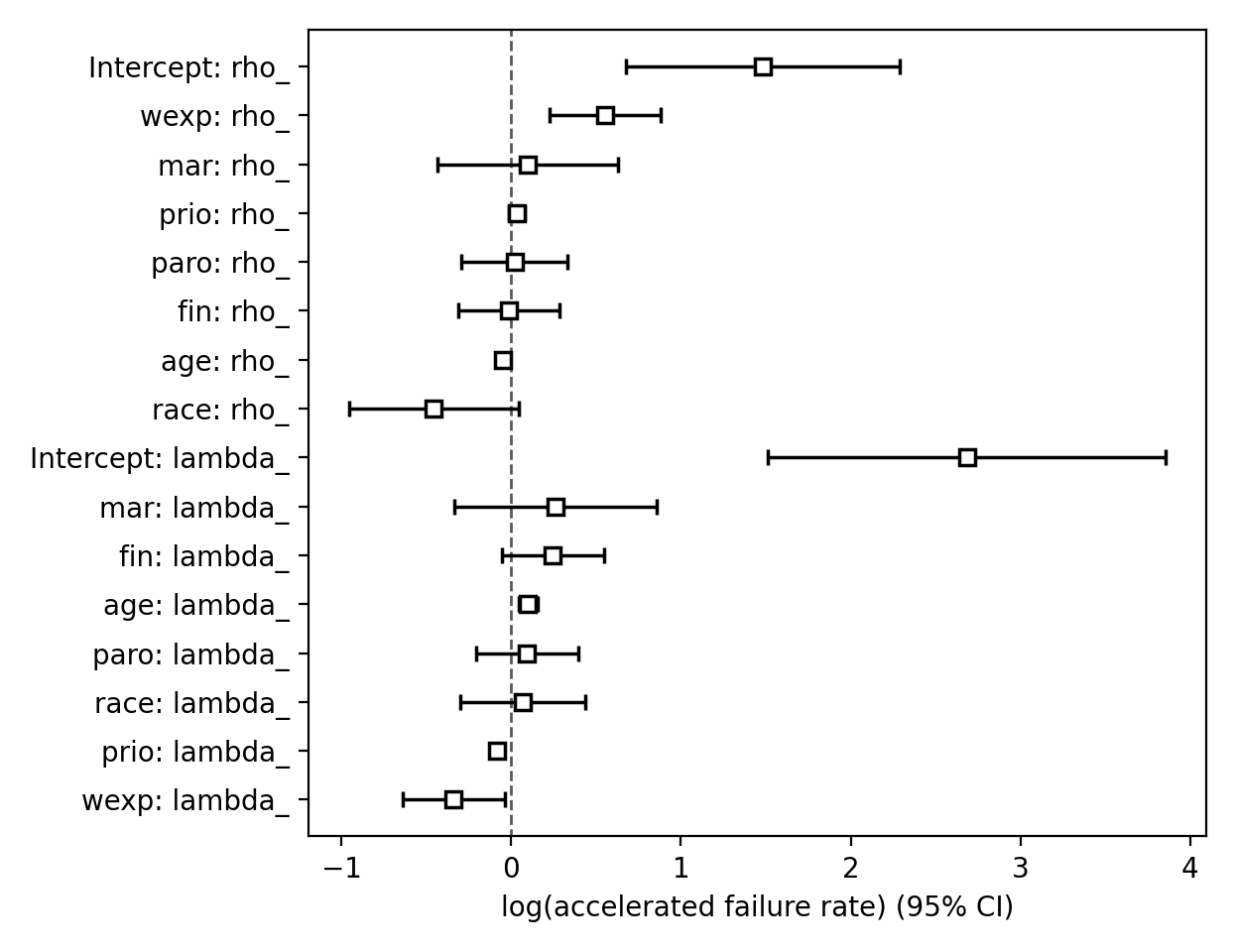We can observe the influence a variable in the model by plotting the outcome (i.e. survival) of changing the variable. This is done using plot_partial_effects_on_outcome(), and this is also a nice time to observe the effects of modeling rho_ vs keeping it fixed. Below we fit the Weibull model to the same dataset twice, but in the first model we model rho_ and in the second model we don’t. We when vary the prio (which is the number of prior arrests) and observe how the survival changes.

Note

Prior to lifelines v0.25.0, this method used to be called plot_covariate_group. It’s been renamed to plot_partial_effects_on_outcome (a much clearer name, I hope).

fig, ax = plt.subplots(nrows=1, ncols=2, figsize=(10, 4))

times = np.arange(0, 100)
wft_model_rho = WeibullAFTFitter().fit(rossi, 'week', 'arrest', ancillary=True, timeline=times)
wft_model_rho.plot_partial_effects_on_outcome('prio', range(0, 16, 3), cmap='coolwarm', ax=ax)
ax.set_title("Modelling rho_")

wft_not_model_rho = WeibullAFTFitter().fit(rossi, 'week', 'arrest', ancillary=False, timeline=times)
wft_not_model_rho.plot_partial_effects_on_outcome('prio', range(0, 16, 3), cmap='coolwarm', ax=ax)
ax.set_title("Not modelling rho_");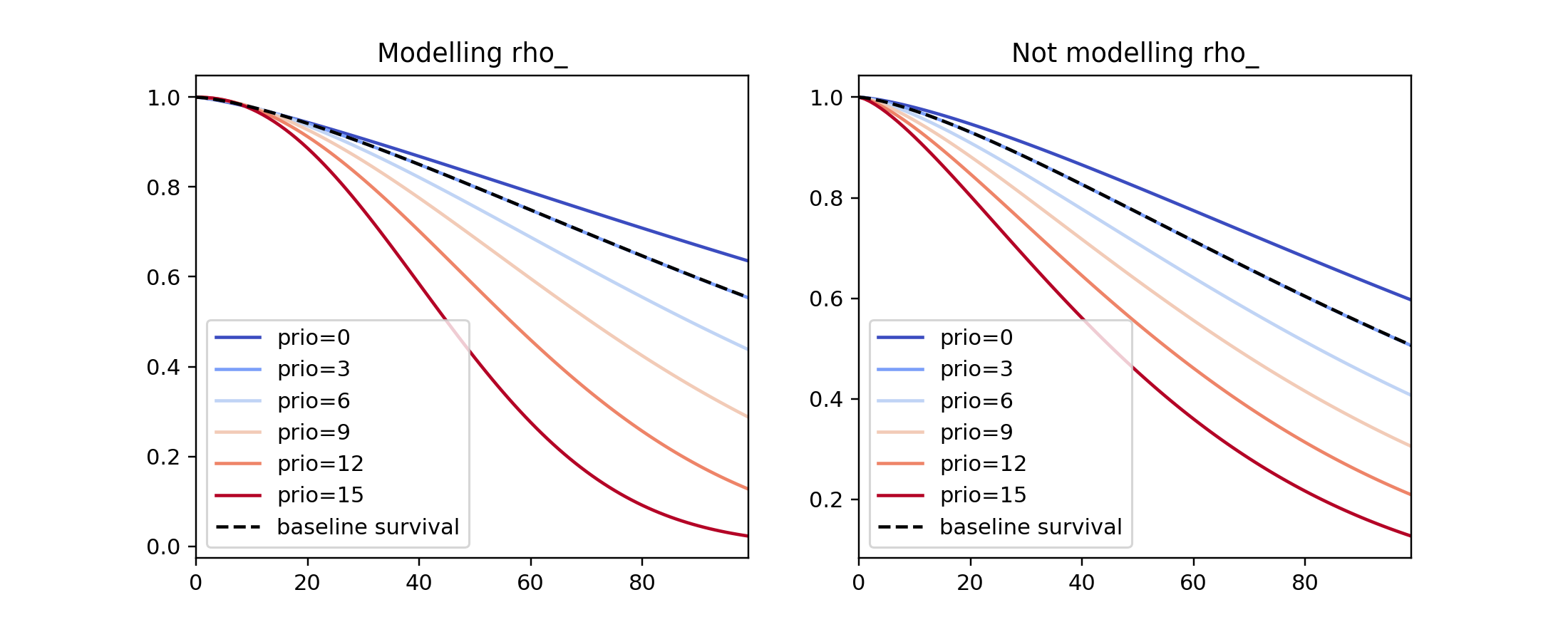Comparing a few of these survival functions side by side, be can see that modeling rho_ produces a more flexible (diverse) set of survival functions.

fig, ax = plt.subplots(nrows=1, ncols=1, figsize=(7, 4))

# modeling rho == solid line
wft_model_rho.plot_partial_effects_on_outcome('prio', range(0, 16, 5), cmap='coolwarm', ax=ax, lw=2, plot_baseline=False)

# not modeling rho == dashed line
wft_not_model_rho.plot_partial_effects_on_outcome('prio', range(0, 16, 5), cmap='coolwarm', ax=ax, ls='--', lw=2, plot_baseline=False)

ax.get_legend().remove()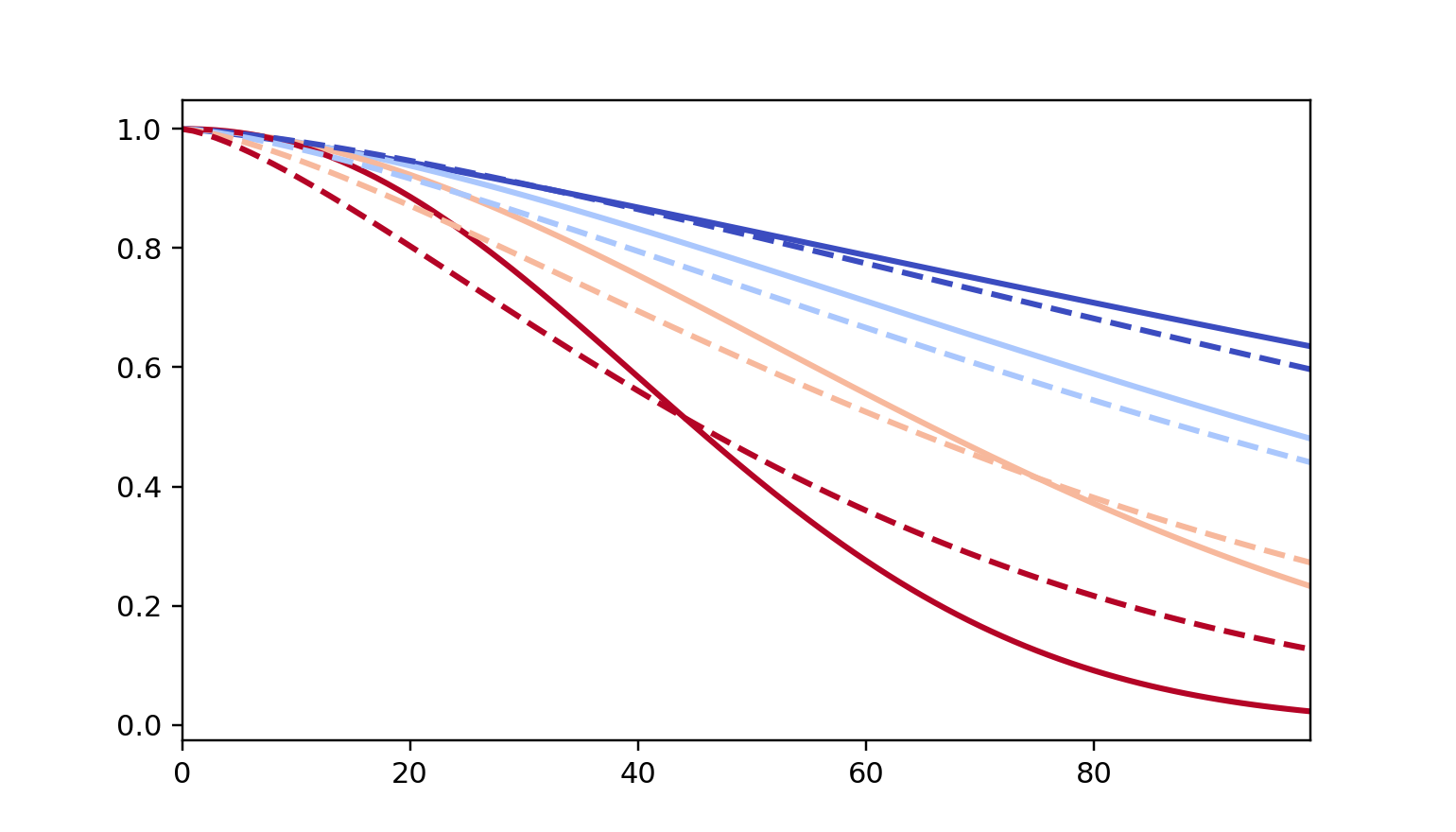You read more about and see other examples of the extensions to in the docs for plot_partial_effects_on_outcome()

### Prediction¶

Given a new subject, we’d like to ask questions about their future survival. When are they likely to experience the event? What does their survival function look like? The WeibullAFTFitter is able to answer these. If we have modeled the ancillary covariates, we are required to include those as well:

X = rossi.loc[:10]

aft.predict_cumulative_hazard(X, ancillary=X)
aft.predict_survival_function(X, ancillary=X)
aft.predict_median(X, ancillary=X)
aft.predict_percentile(X, p=0.9, ancillary=X)
aft.predict_expectation(X, ancillary=X)


There are two hyper-parameters that can be used to to achieve a better test score. These are penalizer and l1_ratio in the call to WeibullAFTFitter. The penalizer is similar to scikit-learn’s ElasticNet model, see their docs. (However, lifelines will also accept an array for custom penalty value per variable, see Cox docs above)

aft_with_elastic_penalty = WeibullAFTFitter(penalizer=1e-4, l1_ratio=1.0)
aft_with_elastic_penalty.fit(rossi, 'week', 'arrest')
aft_with_elastic_penalty.predict_median(rossi)

aft_with_elastic_penalty.print_summary(columns=['coef', 'exp(coef)'])

"""
<lifelines.WeibullAFTFitter: fitted with 432 total observations, 318 right-censored observations>
duration col = 'week'
event col = 'arrest'
penalizer = 0.0001
number of observations = 432
number of events observed = 114
log-likelihood = -679.97
time fit was run = 2020-08-09 15:04:35 UTC

---
coef  exp(coef)
param   covariate
lambda_ age         0.04       1.04
fin         0.27       1.31
mar         0.31       1.36
paro        0.06       1.06
prio       -0.07       0.94
race       -0.22       0.80
wexp        0.11       1.11
Intercept   3.99      54.11
rho_    Intercept   0.34       1.40
---
Concordance = 0.64
AIC = 1377.93
log-likelihood ratio test = 33.31 on 7 df
-log2(p) of ll-ratio test = 15.40

"""


### The log-normal and log-logistic AFT models¶

There are also the LogNormalAFTFitter and LogLogisticAFTFitter models, which instead of assuming that the survival time distribution is Weibull, we assume it is Log-Normal or Log-Logistic, respectively. They have identical APIs to the WeibullAFTFitter, but the parameter names are different.

from lifelines import LogLogisticAFTFitter
from lifelines import LogNormalAFTFitter

llf = LogLogisticAFTFitter().fit(rossi, 'week', 'arrest')
lnf = LogNormalAFTFitter().fit(rossi, 'week', 'arrest')


### More AFT models: CRC model and generalized gamma model¶

For a flexible and smooth parametric model, there is the GeneralizedGammaRegressionFitter. This model is actually a generalization of all the AFT models above (that is, specific values of its parameters represent another model ) - see docs for specific parameter values. The API is slightly different however, and looks more like how custom regression models are built (see next section on Custom Regression Models).

from lifelines import GeneralizedGammaRegressionFitter

df['Intercept'] = 1.

# this will regress df against all 3 parameters
ggf = GeneralizedGammaRegressionFitter(penalizer=1.).fit(df, 'week', 'arrest')
ggf.print_summary()

# If we want fine control over the parameters <-> covariates.
# The values in the dict become can be formulas, or column names in lists:
regressors = {
'mu_': rossi.columns.difference(['arrest', 'week']),
'sigma_': ["age", "Intercept"],
'lambda_': 'age + 1',
}

ggf = GeneralizedGammaRegressionFitter(penalizer=0.0001).fit(df, 'week', 'arrest', regressors=regressors)
ggf.print_summary()


Similarly, there is the CRC model that is uses splines to model the time. See a blog post about it here.

### The piecewise-exponential regression models¶

Another class of parametric models involves more flexible modeling of the hazard function. The PiecewiseExponentialRegressionFitter can model jumps in the hazard (think: the differences in “survival-of-staying-in-school” between 1st year, 2nd year, 3rd year, and 4th year students), and constant values between jumps. The ability to specify when these jumps occur, called breakpoints, offers modelers great flexibility. An example application involving customer churn is available in this notebook.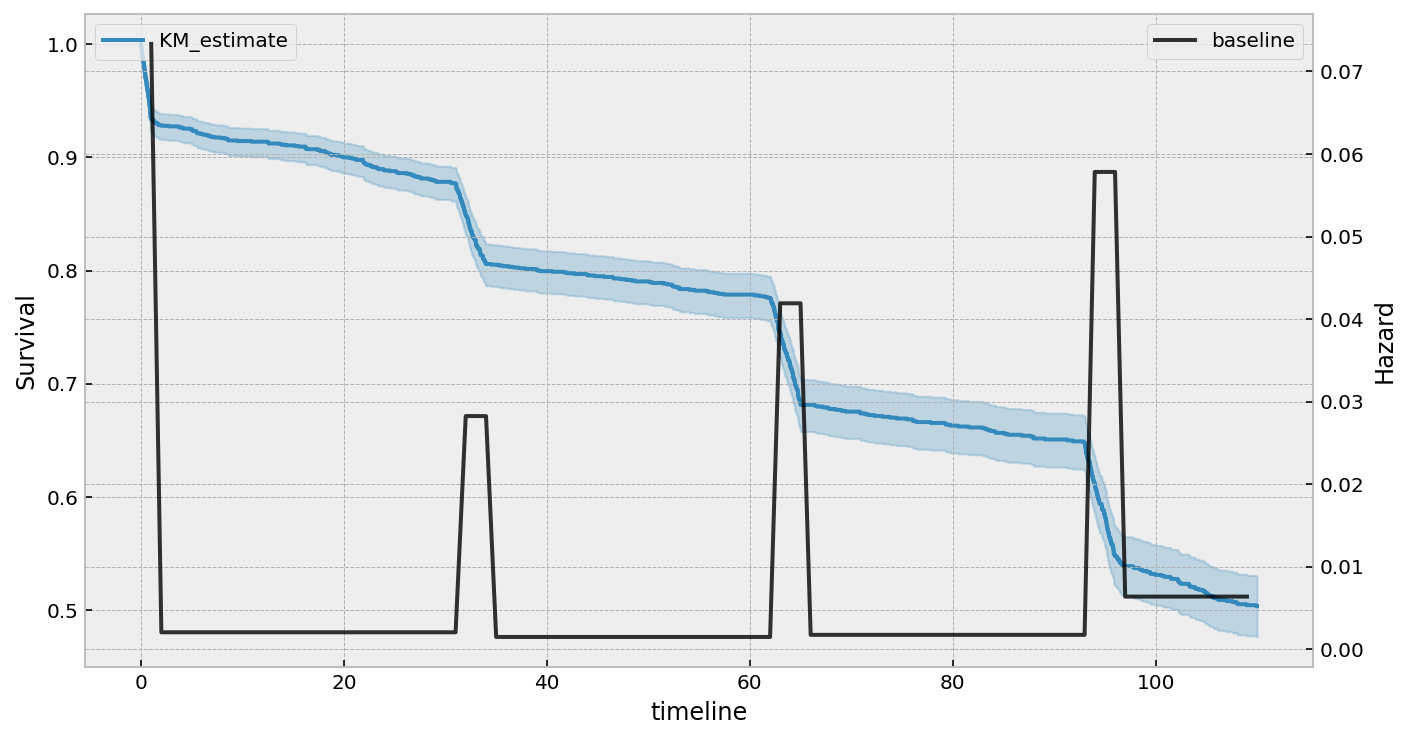### AIC and model selection for parametric models¶

Often, you don’t know a priori which parametric model to use. Each model has some assumptions built-in (not implemented yet in lifelines), but a quick and effective method is to compare the AICs for each fitted model. (In this case, the number of parameters for each model is the same, so really this is comparing the log-likelihood). The model with the smallest AIC does the best job of fitting to the data with a minimal degrees of freedom.

from lifelines import LogLogisticAFTFitter, WeibullAFTFitter, LogNormalAFTFitter

llf = LogLogisticAFTFitter().fit(rossi, 'week', 'arrest')
lnf = LogNormalAFTFitter().fit(rossi, 'week', 'arrest')
wf = WeibullAFTFitter().fit(rossi, 'week', 'arrest')

print(llf.AIC_)  # 1377.877
print(lnf.AIC_)  # 1384.469
print(wf.AIC_)   # 1377.833, slightly the best model.

# with some heterogeneity in the ancillary parameters
ancillary = rossi[['prio']]
llf = LogLogisticAFTFitter().fit(rossi, 'week', 'arrest', ancillary=ancillary)
lnf = LogNormalAFTFitter().fit(rossi, 'week', 'arrest', ancillary=ancillary)
wf = WeibullAFTFitter().fit(rossi, 'week', 'arrest', ancillary=ancillary)

print(llf.AIC_) # 1377.89, the best model here, but not the overall best.
print(lnf.AIC_) # 1380.79
print(wf.AIC_)  # 1379.21


### Left, right and interval censored data¶

The parametric models have APIs that handle left and interval censored data, too. The API for them is different than the API for fitting to right censored data. Here’s an example with interval censored data.

from lifelines.datasets import load_diabetes

df['gender'] = df['gender'] == 'male'

"""
left  right  gender
1    24     27    True
2    22     22   False
3    37     39    True
4    20     20    True
5     1     16    True
"""

wf = WeibullAFTFitter().fit_interval_censoring(df, lower_bound_col='left', upper_bound_col='right')
wf.print_summary()

"""
<lifelines.WeibullAFTFitter: fitted with 731 total observations, 136 interval-censored observations>
lower bound col = 'left'
upper bound col = 'right'
number of observations = 731
number of events observed = 595
log-likelihood = -2027.20
time fit was run = 2020-08-09 15:05:09 UTC

---
coef  exp(coef)   se(coef)   coef lower 95%   coef upper 95%  exp(coef) lower 95%  exp(coef) upper 95%
param   covariate
lambda_ gender      0.05       1.05       0.03            -0.01             0.10                 0.99                 1.10
Intercept   2.91      18.32       0.02             2.86             2.95                17.53                19.14
rho_    Intercept   1.04       2.83       0.03             0.98             1.09                 2.67                 2.99
z      p   -log2(p)
param   covariate
lambda_ gender      1.66   0.10       3.38
Intercept 130.15 <0.005        inf
rho_    Intercept  36.91 <0.005     988.46
---
AIC = 4060.39
log-likelihood ratio test = 2.74 on 1 df
-log2(p) of ll-ratio test = 3.35
"""


Another example of using lifelines for interval censored data is located here.

### Custom parametric regression models¶

lifelines has a very general syntax for creating your own parametric regression models. If you are looking to create your own custom models, see docs Custom Regression Models.

Warning

This implementation is still experimental.

Aalen’s Additive model is another regression model we can use. Like the Cox model, it defines the hazard rate, but instead of the linear model being multiplicative like the Cox model, the Aalen model is additive. Specifically:

$h(t|x) = b_0(t) + b_1(t) x_1 + ... + b_N(t) x_N$

Inference typically does not estimate the individual $$b_i(t)$$ but instead estimates $$\int_0^t b_i(s) \; ds$$ (similar to the estimate of the hazard rate using NelsonAalenFitter). This is important when interpreting plots produced.

For this exercise, we will use the regime dataset and include the categorical variables un_continent_name (eg: Asia, North America,…), the regime type (e.g., monarchy, civilian,…) and the year the regime started in, start_year. The estimator to fit unknown coefficients in Aalen’s additive model is located under AalenAdditiveFitter.

from lifelines import AalenAdditiveFitter


ctryname cowcode2 politycode un_region_name un_continent_name ehead leaderspellreg democracy regime start_year duration observed
Afghanistan 700 700 Southern Asia Asia Mohammad Zahir Shah Mohammad Zahir Shah.Afghanistan.1946.1952.Monarchy Non-democracy Monarchy 1946 7 1
Afghanistan 700 700 Southern Asia Asia Sardar Mohammad Daoud Sardar Mohammad Daoud.Afghanistan.1953.1962.Civilian Dict Non-democracy Civilian Dict 1953 10 1
Afghanistan 700 700 Southern Asia Asia Mohammad Zahir Shah Mohammad Zahir Shah.Afghanistan.1963.1972.Monarchy Non-democracy Monarchy 1963 10 1
Afghanistan 700 700 Southern Asia Asia Sardar Mohammad Daoud Sardar Mohammad Daoud.Afghanistan.1973.1977.Civilian Dict Non-democracy Civilian Dict 1973 5 0
Afghanistan 700 700 Southern Asia Asia Nur Mohammad Taraki Nur Mohammad Taraki.Afghanistan.1978.1978.Civilian Dict Non-democracy Civilian Dict 1978 1 0

We have also included the coef_penalizer option. During the estimation, a linear regression is computed at each step. Often the regression can be unstable (due to high co-linearity or small sample sizes) – adding a penalizer term controls the stability. I recommend always starting with a small penalizer term – if the estimates still appear to be too unstable, try increasing it.

aaf = AalenAdditiveFitter(coef_penalizer=1.0, fit_intercept=False)


An instance of AalenAdditiveFitter includes a fit() method that performs the inference on the coefficients. This method accepts a pandas DataFrame: each row is an individual and columns are the covariates and two individual columns: a duration column and a boolean event occurred column (where event occurred refers to the event of interest - expulsion from government in this case)

df['T'] = data['duration']
df['E'] = data['observed']

aaf.fit(df, 'T', event_col='E', formula='un_continent_name + regime + start_year')


After fitting, the instance exposes a cumulative_hazards_ DataFrame containing the estimates of $$\int_0^t b_i(s) \; ds$$:

aaf.cumulative_hazards_.head()

baseline un_continent_name[T.Americas] un_continent_name[T.Asia] un_continent_name[T.Europe] un_continent_name[T.Oceania] regime[T.Military Dict] regime[T.Mixed Dem] regime[T.Monarchy] regime[T.Parliamentary Dem] regime[T.Presidential Dem] start_year
-0.03447 -0.03173 0.06216 0.2058 -0.009559 0.07611 0.08729 -0.1362 0.04885 0.1285 0.000092
0.14278 -0.02496 0.11122 0.2083 -0.079042 0.11704 0.36254 -0.2293 0.17103 0.1238 0.000044
0.30153 -0.07212 0.10929 0.1614 0.063030 0.16553 0.68693 -0.2738 0.33300 0.1499 0.000004
0.37969 0.06853 0.15162 0.2609 0.185569 0.22695 0.95016 -0.2961 0.37351 0.4311 -0.000032
0.36749 0.20201 0.21252 0.2429 0.188740 0.25127 1.15132 -0.3926 0.54952 0.7593 -0.000000

AalenAdditiveFitter also has built in plotting:

aaf.plot(columns=['regime[T.Presidential Dem]', 'Intercept', 'un_continent_name[T.Europe]'], iloc=slice(1,15))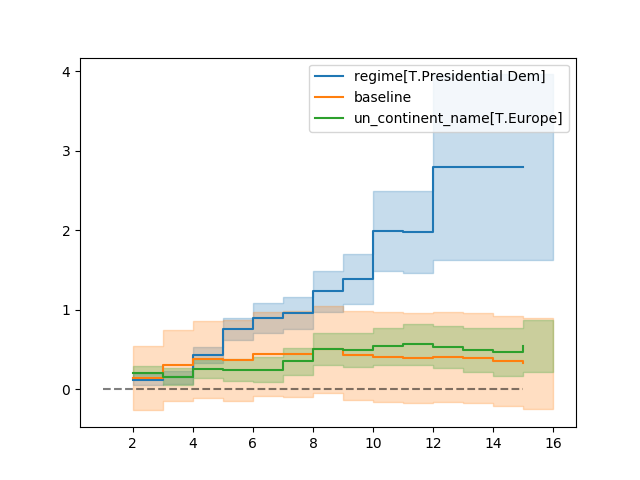Regression is most interesting if we use it on data we have not yet seen, i.e., prediction! We can use what we have learned to predict individual hazard rates, survival functions, and median survival time. The dataset we are using is available up until 2008, so let’s use this data to predict the duration of former Canadian Prime Minister Stephen Harper.

ix = (data['ctryname'] == 'Canada') & (data['start_year'] == 2006)
harper = df.loc[ix]
print("Harper's unique data point:")
print(harper)

Harper's unique data point:
baseline  un_continent_name[T.Americas]  un_continent_name[T.Asia] ...  start_year  T  E
268       1.0                            1.0                        0.0 ...      2006.0  3  0

ax = plt.subplot(2,1,1)
aaf.predict_cumulative_hazard(harper).plot(ax=ax)

ax = plt.subplot(2,1,2)
aaf.predict_survival_function(harper).plot(ax=ax);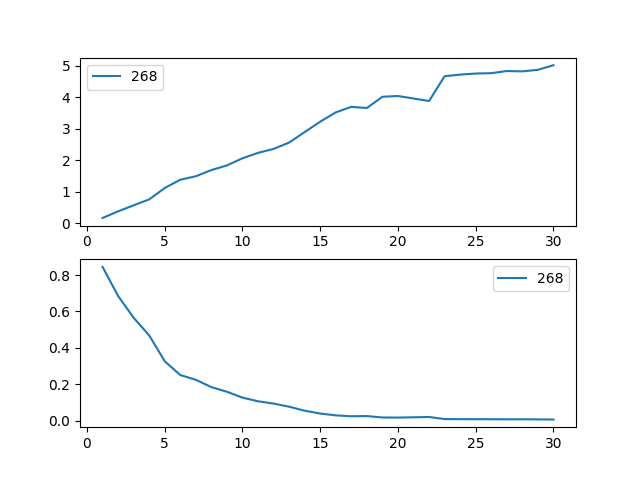Note

Because of the nature of the model, estimated survival functions of individuals can increase. This is an expected artifact of Aalen’s additive model.

## Model selection and calibration in survival regression¶

### Parametric vs semi-parametric models¶

Above, we’ve displayed two semi-parametric models (Cox model and Aalen’s model), and a family of parametric models. Which should you choose? What are the advantages and disadvantages of either? I suggest reading the two following StackExchange answers to get a better idea of what experts think:

### Model selection based on residuals¶

The sections Testing the Proportional Hazard Assumptions and Assessing Cox model fit using residuals may be useful for modeling your data better.

Note

Work is being done to extend residual methods to all regression models. Stay tuned.

### Model selection based on predictive power and fit¶

If censoring is present, it’s not appropriate to use a loss function like mean-squared-error or mean-absolute-loss. This is because the difference between a censored value and the predicted value could be due to poor prediction or due to censoring. Below we introduce alternative ways to measure prediction performance.

#### Log-likelihood¶

In this author’s opinion, the best way to measure predictive performance is evaluating the log-likelihood on out-of-sample data. The log-likelihood correctly handles any type of censoring, and is precisely what we are maximizing in the model training. The in-sample log-likelihood is available under log_likelihood_ of any regression model. For out-of-sample data, the score() method (available on all regression models) can be used. This returns the average evaluation of the out-of-sample log-likelihood. We want to maximize this.

from lifelines import CoxPHFitter

rossi = load_rossi().sample(frac=1.0, random_state=25)  # ensures the reproducibility of the example
train_rossi = rossi.iloc[:400]
test_rossi = rossi.iloc[400:]

cph_l1 = CoxPHFitter(penalizer=0.1, l1_ratio=1.).fit(train_rossi, 'week', 'arrest')
cph_l2 = CoxPHFitter(penalizer=0.1, l1_ratio=0.).fit(train_rossi, 'week', 'arrest')

print(cph_l1.score(test_rossi))
print(cph_l2.score(test_rossi))  # higher is better


#### Akaike information criterion (AIC)¶

For within-sample validation, the AIC is a great metric for comparing models as it relies on the log-likelihood. It’s available under AIC_ for parametric models, and AIC_partial_ for Cox models (because the Cox model maximizes a partial log-likelihood, it can’t be reliably compared to parametric model’s AIC.)

from lifelines import CoxPHFitter

cph_l2 = CoxPHFitter(penalizer=0.1, l1_ratio=0.).fit(rossi, 'week', 'arrest')
cph_l1 = CoxPHFitter(penalizer=0.1, l1_ratio=1.).fit(rossi, 'week', 'arrest')

print(cph_l2.AIC_partial_) # lower is better
print(cph_l1.AIC_partial_)


#### Concordance Index¶

Another censoring-sensitive measure is the concordance-index, also known as the c-index. This measure evaluates the accuracy of the ranking of predicted time. It is in fact a generalization of AUC, another common loss function, and is interpreted similarly:

• 0.5 is the expected result from random predictions,
• 1.0 is perfect concordance and,
• 0.0 is perfect anti-concordance (multiply predictions with -1 to get 1.0)

Here is an excellent introduction & description of the c-index for new users.

Fitted survival models typically have a concordance index between 0.55 and 0.75 (this may seem bad, but even a perfect model has a lot of noise than can make a high score impossible). In lifelines, a fitted model’s concordance-index is present in the output of score(), but also available under the concordance_index_ property. Generally, the measure is implemented in lifelines under lifelines.utils.concordance_index() and accepts the actual times (along with any censored subjects) and the predicted times.

from lifelines import CoxPHFitter

cph = CoxPHFitter()
cph.fit(rossi, duration_col="week", event_col="arrest")

# fours ways to view the c-index:
# method one
cph.print_summary()

# method two
print(cph.concordance_index_)

# method three
print(cph.score(rossi, scoring_method="concordance_index"))

# method four
from lifelines.utils import concordance_index
print(concordance_index(rossi['week'], -cph.predict_partial_hazard(rossi), rossi['arrest']))


Note

Remember, the concordance score evaluates the relative rankings of subject’s event times. Thus, it is scale and shift invariant (i.e. you can multiple by a positive constant, or add a constant, and the rankings won’t change). A model maximized for concordance-index does not necessarily give good predicted times, but will give good predicted rankings.

#### Cross validation¶

lifelines has an implementation of k-fold cross validation under lifelines.utils.k_fold_cross_validation(). This function accepts an instance of a regression fitter (either CoxPHFitter of AalenAdditiveFitter), a dataset, plus k (the number of folds to perform, default 5). On each fold, it splits the data into a training set and a testing set fits itself on the training set and evaluates itself on the testing set (using the concordance measure by default).

from lifelines import CoxPHFitter
from lifelines.utils import k_fold_cross_validation

cph = CoxPHFitter()
scores = k_fold_cross_validation(cph, regression_dataset, 'T', event_col='E', k=3)
print(scores)
#[-2.9896, -3.08810, -3.02747]

scores = k_fold_cross_validation(cph, regression_dataset, 'T', event_col='E', k=3, scoring_method="concordance_index")
print(scores)
# [0.5449, 0.5587, 0.6179]


Also, lifelines has wrappers for compatibility with scikit learn for making cross-validation and grid-search even easier.

### Model probability calibration¶

New in lifelines v0.24.11 is the survival_probability_calibration() function to measure your fitted survival model against observed frequencies of events. We follow the advice in “Graphical calibration curves and the integrated calibration index (ICI) for survival models” by P. Austin and co., and use create a smoothed calibration curve using a flexible spline regression model (this avoids the traditional problem of binning the continuous-valued probability, and handles censored data).

from lifelines import CoxPHFitter
from lifelines.calibration import survival_probability_calibration

cph = CoxPHFitter(baseline_estimation_method="spline", n_baseline_knots=3)
cph.fit(rossi, "week", "arrest")

survival_probability_calibration(cph, rossi, t0=25)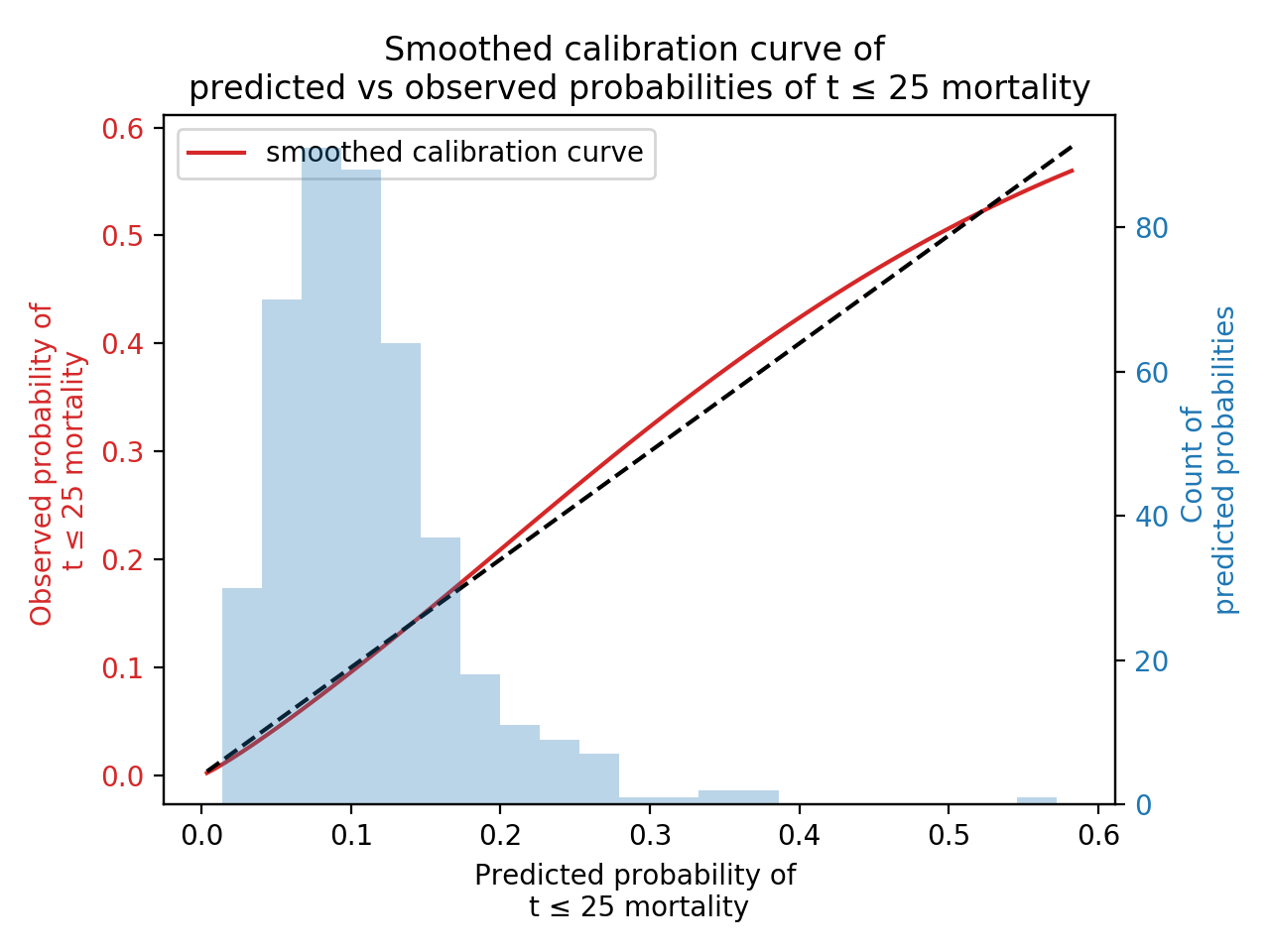## Prediction on censored subjects¶

A common use case is to predict the event time of censored subjects. This is easy to do, but we first have to calculate an important conditional probability. Let $$T$$ be the (random) event time for some subject, and $$S(t)≔P(T > t)$$ be their survival function. We are interested in answering the following: What is a subject’s new survival function given I know the subject has lived past time :math:s? Mathematically:

\begin{split}\begin{align*} P(T > t \;|\; T > s) &= \frac{P(T > t \;\text{and}\; T > s)}{P(T > s)} \\ &= \frac{P(T > t)}{P(T > s)} \\ &= \frac{S(t)}{S(s)} \end{align*}\end{split}

Thus we scale the original survival function by the survival function at time $$s$$ (everything prior to $$s$$ should be mapped to 1.0 as well, since we are working with probabilities and we know that the subject was alive before $$s$$).

This is such a common calculation that lifelines has all this built in. The conditional_after kwarg in all prediction methods allows you to specify what $$s$$ is per subject. Below we predict the remaining life of censored subjects:

# all regression models can be used here, WeibullAFTFitter is used for illustration
wf = WeibullAFTFitter().fit(rossi, "week", "arrest")

# filter down to just censored subjects to predict remaining survival
censored_subjects = rossi.loc[~rossi['arrest'].astype(bool)]
censored_subjects_last_obs = censored_subjects['week']

# predict new survival function
wf.predict_survival_function(censored_subjects, conditional_after=censored_subjects_last_obs)

# predict median remaining life
wf.predict_median(censored_subjects, conditional_after=censored_subjects_last_obs)


Note

It’s important to remember that this is now computing a conditional probability (or metric), so if the result of predict_median is 10.5, then the entire lifetime is 10.5 + conditional_after.

Note

If using conditional_after to predict on uncensored subjects, then conditional_after should probably be set to 0, or left blank.